# lecture-a-java-review.ppt

Jun. 1, 2023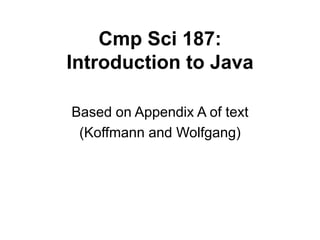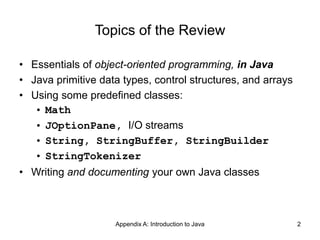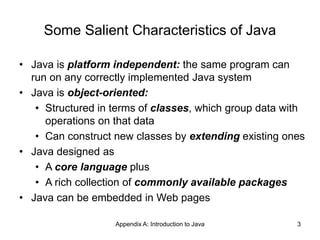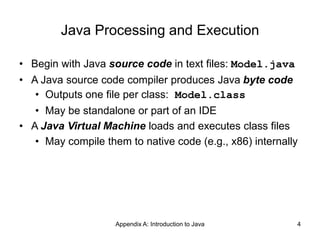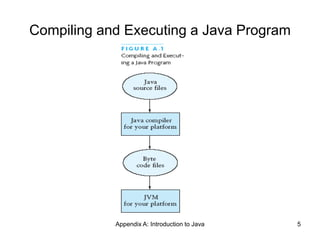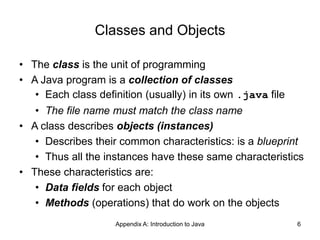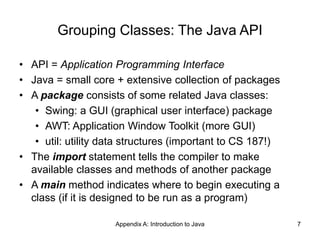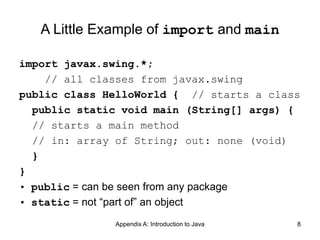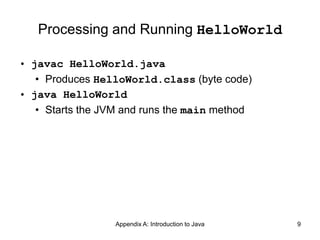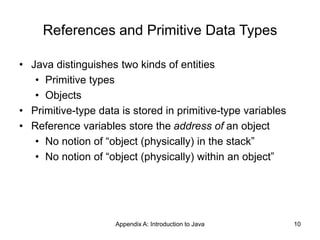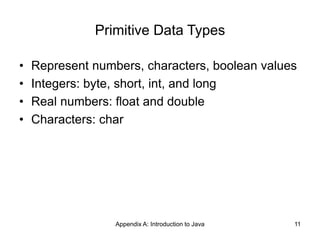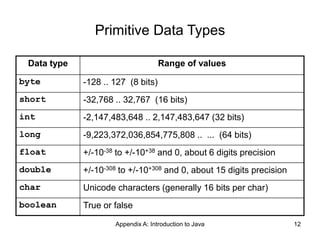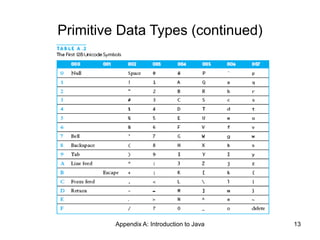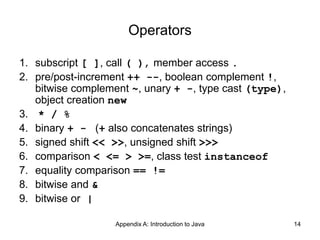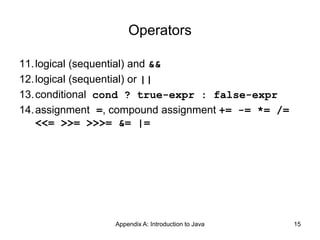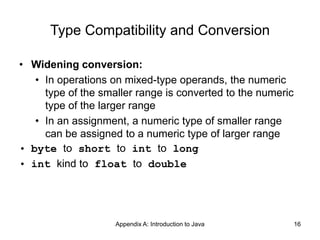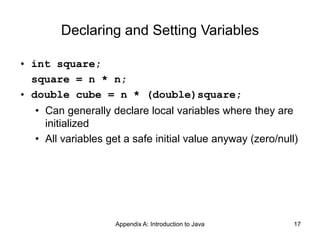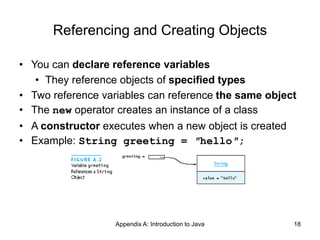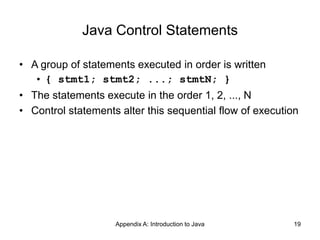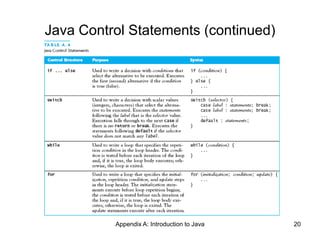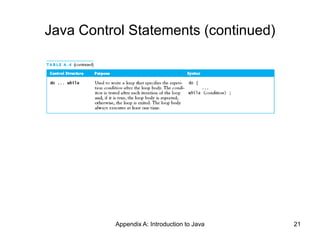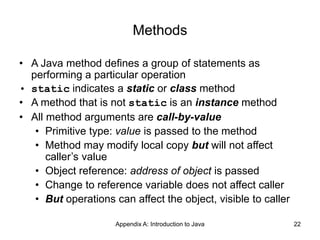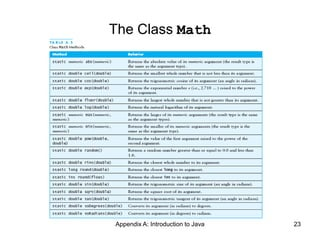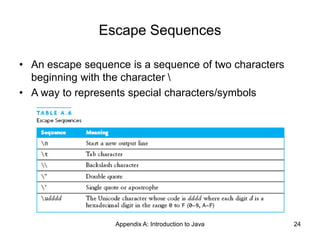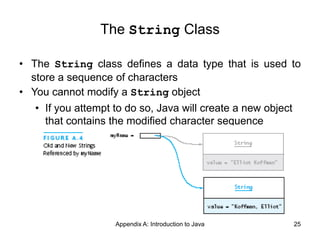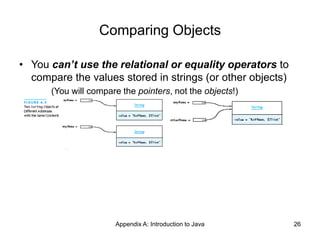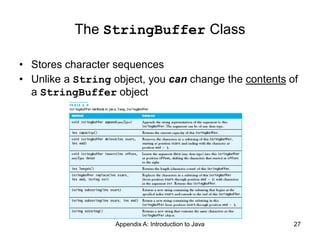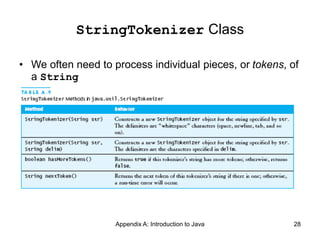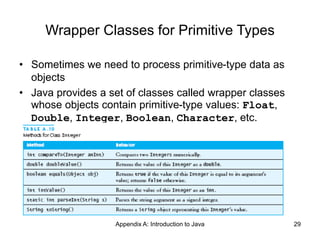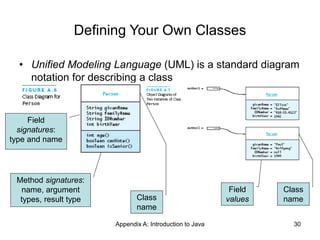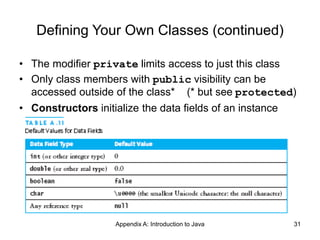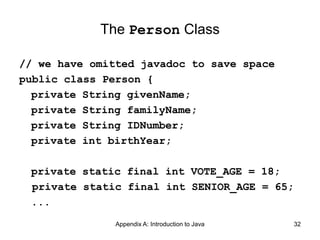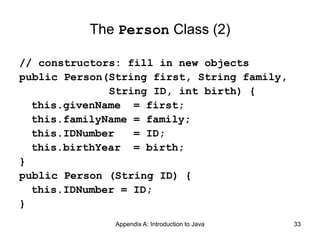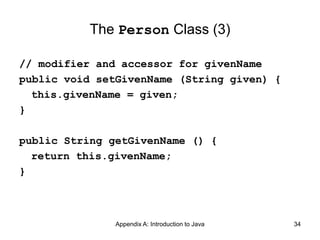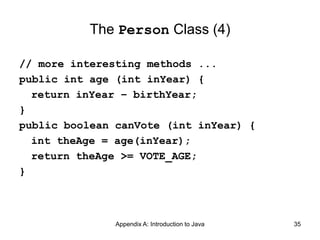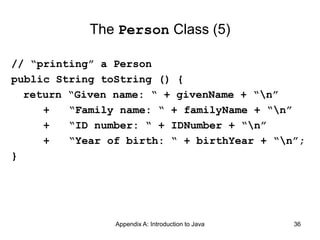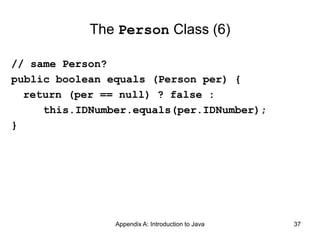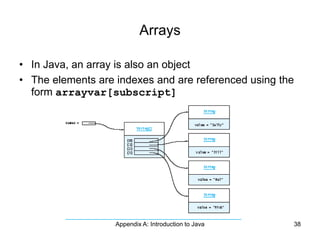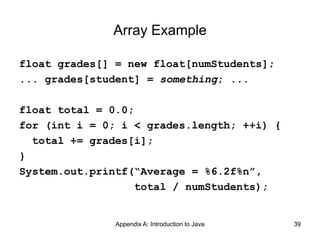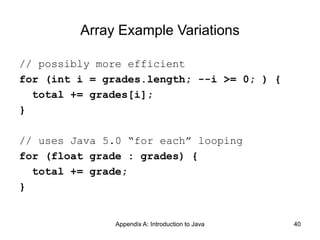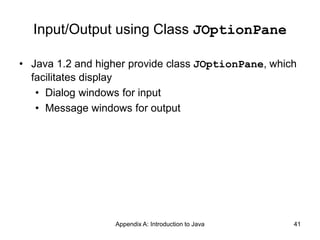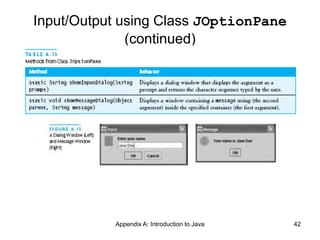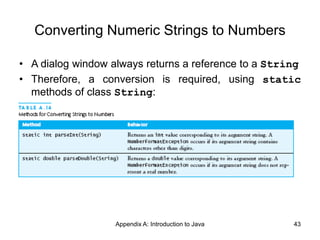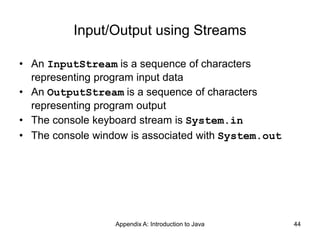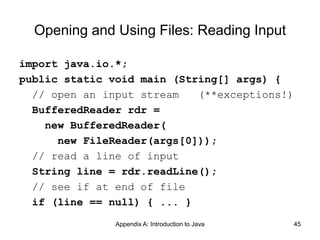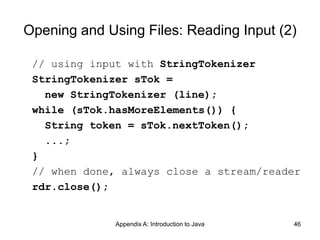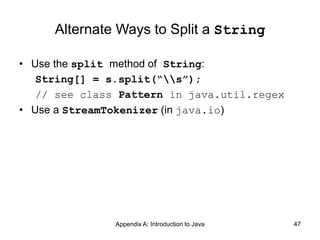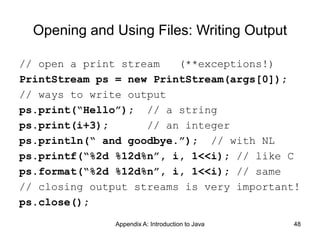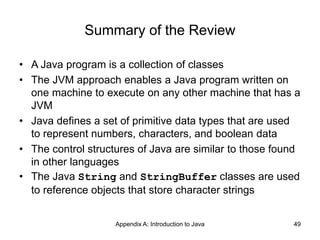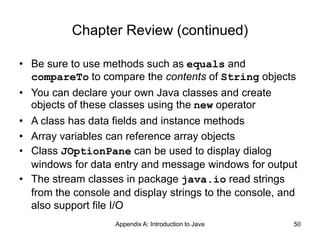1 of 50

### lecture-a-java-review.ppt

• 1. Cmp Sci 187: Introduction to Java Based on Appendix A of text (Koffmann and Wolfgang)
• 2. Appendix A: Introduction to Java 2 Topics of the Review • Essentials of object-oriented programming, in Java • Java primitive data types, control structures, and arrays • Using some predefined classes: • Math • JOptionPane, I/O streams • String, StringBuffer, StringBuilder • StringTokenizer • Writing and documenting your own Java classes
• 3. Appendix A: Introduction to Java 3 Some Salient Characteristics of Java • Java is platform independent: the same program can run on any correctly implemented Java system • Java is object-oriented: • Structured in terms of classes, which group data with operations on that data • Can construct new classes by extending existing ones • Java designed as • A core language plus • A rich collection of commonly available packages • Java can be embedded in Web pages
• 4. Appendix A: Introduction to Java 4 Java Processing and Execution • Begin with Java source code in text files: Model.java • A Java source code compiler produces Java byte code • Outputs one file per class: Model.class • May be standalone or part of an IDE • A Java Virtual Machine loads and executes class files • May compile them to native code (e.g., x86) internally
• 5. Appendix A: Introduction to Java 5 Compiling and Executing a Java Program
• 6. Appendix A: Introduction to Java 6 Classes and Objects • The class is the unit of programming • A Java program is a collection of classes • Each class definition (usually) in its own .java file • The file name must match the class name • A class describes objects (instances) • Describes their common characteristics: is a blueprint • Thus all the instances have these same characteristics • These characteristics are: • Data fields for each object • Methods (operations) that do work on the objects
• 7. Appendix A: Introduction to Java 7 Grouping Classes: The Java API • API = Application Programming Interface • Java = small core + extensive collection of packages • A package consists of some related Java classes: • Swing: a GUI (graphical user interface) package • AWT: Application Window Toolkit (more GUI) • util: utility data structures (important to CS 187!) • The import statement tells the compiler to make available classes and methods of another package • A main method indicates where to begin executing a class (if it is designed to be run as a program)
• 8. Appendix A: Introduction to Java 8 A Little Example of import and main import javax.swing.*; // all classes from javax.swing public class HelloWorld { // starts a class public static void main (String[] args) { // starts a main method // in: array of String; out: none (void) } } • public = can be seen from any package • static = not “part of” an object
• 9. Appendix A: Introduction to Java 9 Processing and Running HelloWorld • javac HelloWorld.java • Produces HelloWorld.class (byte code) • java HelloWorld • Starts the JVM and runs the main method
• 10. Appendix A: Introduction to Java 10 References and Primitive Data Types • Java distinguishes two kinds of entities • Primitive types • Objects • Primitive-type data is stored in primitive-type variables • Reference variables store the address of an object • No notion of “object (physically) in the stack” • No notion of “object (physically) within an object”
• 11. Appendix A: Introduction to Java 11 Primitive Data Types • Represent numbers, characters, boolean values • Integers: byte, short, int, and long • Real numbers: float and double • Characters: char
• 12. Appendix A: Introduction to Java 12 Primitive Data Types Data type Range of values byte -128 .. 127 (8 bits) short -32,768 .. 32,767 (16 bits) int -2,147,483,648 .. 2,147,483,647 (32 bits) long -9,223,372,036,854,775,808 .. ... (64 bits) float +/-10-38 to +/-10+38 and 0, about 6 digits precision double +/-10-308 to +/-10+308 and 0, about 15 digits precision char Unicode characters (generally 16 bits per char) boolean True or false
• 13. Appendix A: Introduction to Java 13 Primitive Data Types (continued)
• 14. Appendix A: Introduction to Java 14 Operators 1. subscript [ ], call ( ), member access . 2. pre/post-increment ++ --, boolean complement !, bitwise complement ~, unary + -, type cast (type), object creation new 3. * / % 4. binary + - (+ also concatenates strings) 5. signed shift << >>, unsigned shift >>> 6. comparison < <= > >=, class test instanceof 7. equality comparison == != 8. bitwise and & 9. bitwise or |
• 15. Appendix A: Introduction to Java 15 Operators 11.logical (sequential) and && 12.logical (sequential) or || 13.conditional cond ? true-expr : false-expr 14.assignment =, compound assignment += -= *= /= <<= >>= >>>= &= |=
• 16. Appendix A: Introduction to Java 16 Type Compatibility and Conversion • Widening conversion: • In operations on mixed-type operands, the numeric type of the smaller range is converted to the numeric type of the larger range • In an assignment, a numeric type of smaller range can be assigned to a numeric type of larger range • byte to short to int to long • int kind to float to double
• 17. Appendix A: Introduction to Java 17 Declaring and Setting Variables • int square; square = n * n; • double cube = n * (double)square; • Can generally declare local variables where they are initialized • All variables get a safe initial value anyway (zero/null)
• 18. Appendix A: Introduction to Java 18 Referencing and Creating Objects • You can declare reference variables • They reference objects of specified types • Two reference variables can reference the same object • The new operator creates an instance of a class • A constructor executes when a new object is created • Example: String greeting = ″hello″;
• 19. Appendix A: Introduction to Java 19 Java Control Statements • A group of statements executed in order is written • { stmt1; stmt2; ...; stmtN; } • The statements execute in the order 1, 2, ..., N • Control statements alter this sequential flow of execution
• 20. Appendix A: Introduction to Java 20 Java Control Statements (continued)
• 21. Appendix A: Introduction to Java 21 Java Control Statements (continued)
• 22. Appendix A: Introduction to Java 22 Methods • A Java method defines a group of statements as performing a particular operation • static indicates a static or class method • A method that is not static is an instance method • All method arguments are call-by-value • Primitive type: value is passed to the method • Method may modify local copy but will not affect caller’s value • Object reference: address of object is passed • Change to reference variable does not affect caller • But operations can affect the object, visible to caller
• 23. Appendix A: Introduction to Java 23 The Class Math
• 24. Appendix A: Introduction to Java 24 Escape Sequences • An escape sequence is a sequence of two characters beginning with the character • A way to represents special characters/symbols
• 25. Appendix A: Introduction to Java 25 The String Class • The String class defines a data type that is used to store a sequence of characters • You cannot modify a String object • If you attempt to do so, Java will create a new object that contains the modified character sequence
• 26. Appendix A: Introduction to Java 26 Comparing Objects • You can’t use the relational or equality operators to compare the values stored in strings (or other objects) (You will compare the pointers, not the objects!)
• 27. Appendix A: Introduction to Java 27 The StringBuffer Class • Stores character sequences • Unlike a String object, you can change the contents of a StringBuffer object
• 28. Appendix A: Introduction to Java 28 StringTokenizer Class • We often need to process individual pieces, or tokens, of a String
• 29. Appendix A: Introduction to Java 29 Wrapper Classes for Primitive Types • Sometimes we need to process primitive-type data as objects • Java provides a set of classes called wrapper classes whose objects contain primitive-type values: Float, Double, Integer, Boolean, Character, etc.
• 30. Appendix A: Introduction to Java 30 Defining Your Own Classes • Unified Modeling Language (UML) is a standard diagram notation for describing a class Class name Field values Class name Field signatures: type and name Method signatures: name, argument types, result type
• 31. Appendix A: Introduction to Java 31 Defining Your Own Classes (continued) • The modifier private limits access to just this class • Only class members with public visibility can be accessed outside of the class* (* but see protected) • Constructors initialize the data fields of an instance
• 32. Appendix A: Introduction to Java 32 The Person Class // we have omitted javadoc to save space public class Person { private String givenName; private String familyName; private String IDNumber; private int birthYear; private static final int VOTE_AGE = 18; private static final int SENIOR_AGE = 65; ...
• 33. Appendix A: Introduction to Java 33 The Person Class (2) // constructors: fill in new objects public Person(String first, String family, String ID, int birth) { this.givenName = first; this.familyName = family; this.IDNumber = ID; this.birthYear = birth; } public Person (String ID) { this.IDNumber = ID; }
• 34. Appendix A: Introduction to Java 34 The Person Class (3) // modifier and accessor for givenName public void setGivenName (String given) { this.givenName = given; } public String getGivenName () { return this.givenName; }
• 35. Appendix A: Introduction to Java 35 The Person Class (4) // more interesting methods ... public int age (int inYear) { return inYear – birthYear; } public boolean canVote (int inYear) { int theAge = age(inYear); return theAge >= VOTE_AGE; }
• 36. Appendix A: Introduction to Java 36 The Person Class (5) // “printing” a Person public String toString () { return “Given name: “ + givenName + “n” + “Family name: “ + familyName + “n” + “ID number: “ + IDNumber + “n” + “Year of birth: “ + birthYear + “n”; }
• 37. Appendix A: Introduction to Java 37 The Person Class (6) // same Person? public boolean equals (Person per) { return (per == null) ? false : this.IDNumber.equals(per.IDNumber); }
• 38. Appendix A: Introduction to Java 38 Arrays • In Java, an array is also an object • The elements are indexes and are referenced using the form arrayvar[subscript]
• 39. Appendix A: Introduction to Java 39 Array Example float grades[] = new float[numStudents]; ... grades[student] = something; ... float total = 0.0; for (int i = 0; i < grades.length; ++i) { total += grades[i]; } System.out.printf(“Average = %6.2f%n”, total / numStudents);
• 40. Appendix A: Introduction to Java 40 Array Example Variations // possibly more efficient for (int i = grades.length; --i >= 0; ) { total += grades[i]; } // uses Java 5.0 “for each” looping for (float grade : grades) { total += grade; }
• 41. Appendix A: Introduction to Java 41 Input/Output using Class JOptionPane • Java 1.2 and higher provide class JOptionPane, which facilitates display • Dialog windows for input • Message windows for output
• 42. Appendix A: Introduction to Java 42 Input/Output using Class JOptionPane (continued)
• 43. Appendix A: Introduction to Java 43 Converting Numeric Strings to Numbers • A dialog window always returns a reference to a String • Therefore, a conversion is required, using static methods of class String:
• 44. Appendix A: Introduction to Java 44 Input/Output using Streams • An InputStream is a sequence of characters representing program input data • An OutputStream is a sequence of characters representing program output • The console keyboard stream is System.in • The console window is associated with System.out
• 45. Appendix A: Introduction to Java 45 Opening and Using Files: Reading Input import java.io.*; public static void main (String[] args) { // open an input stream (**exceptions!) BufferedReader rdr = new BufferedReader( new FileReader(args)); // read a line of input String line = rdr.readLine(); // see if at end of file if (line == null) { ... }
• 46. Appendix A: Introduction to Java 46 Opening and Using Files: Reading Input (2) // using input with StringTokenizer StringTokenizer sTok = new StringTokenizer (line); while (sTok.hasMoreElements()) { String token = sTok.nextToken(); ...; } // when done, always close a stream/reader rdr.close();
• 47. Appendix A: Introduction to Java 47 Alternate Ways to Split a String • Use the split method of String: String[] = s.split(“s”); // see class Pattern in java.util.regex • Use a StreamTokenizer (in java.io)
• 48. Appendix A: Introduction to Java 48 Opening and Using Files: Writing Output // open a print stream (**exceptions!) PrintStream ps = new PrintStream(args); // ways to write output ps.print(“Hello”); // a string ps.print(i+3); // an integer ps.println(“ and goodbye.”); // with NL ps.printf(“%2d %12d%n”, i, 1<<i); // like C ps.format(“%2d %12d%n”, i, 1<<i); // same // closing output streams is very important! ps.close();
• 49. Appendix A: Introduction to Java 49 Summary of the Review • A Java program is a collection of classes • The JVM approach enables a Java program written on one machine to execute on any other machine that has a JVM • Java defines a set of primitive data types that are used to represent numbers, characters, and boolean data • The control structures of Java are similar to those found in other languages • The Java String and StringBuffer classes are used to reference objects that store character strings
• 50. Appendix A: Introduction to Java 50 Chapter Review (continued) • Be sure to use methods such as equals and compareTo to compare the contents of String objects • You can declare your own Java classes and create objects of these classes using the new operator • A class has data fields and instance methods • Array variables can reference array objects • Class JOptionPane can be used to display dialog windows for data entry and message windows for output • The stream classes in package java.io read strings from the console and display strings to the console, and also support file I/O